# Mole Fraction Formula

Mole Fraction Formula

Mole fraction is another way of expressing the concentration of a solution or mixture. It is equal to the moles of one component divided by the total moles in the solution or mixture.a = the component that is being identified for mole fraction

Mole fraction is used in a variety of calculations, but most notably for calculating partial pressures.

Mole Fraction Formula Questions:

1. What is the mole fraction of carbon tetrachloride (CCl4) in solution if 3.47 moles of CCl4 is dissolved in 8.54 moles of benzene (C6H6)?

Answer: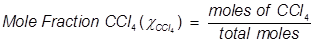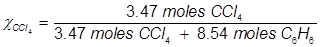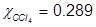2. What is the mole fraction of formaldehyde (CH2O) in solution if 25.7 grams of CH2O is dissolved in 3.25 moles of carbon tetrachloride (CCl4)?

Answer:

The grams of acetone will need to be converted into moles in order to solve for mole fraction.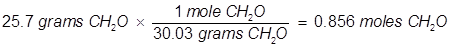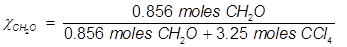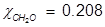Related Links: Unit Vector Formula Mole-Mass Conversions Quiz Mole Ratios Quiz Mole Facts